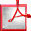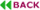Reverse Engineering of Irreducible Polynomials in GF(2m) Arithmetic

Cunxi Yua, Daniel Holcombb and Maciej Ciesielskic
ECE Department, University of Massachusetts, Amherst, USA.
aycunxi@umass.edu
bholcomb@engin.umass.edu
cciesiel@ecs.umass.edu

ABSTRACT

Current techniques for formally verifying circuits implemented in Galois field (GF) arithmetic are limited to those with a known irreducible polynomial P(x). This paper presents a computer algebra based technique that extracts the irreducible polynomial P(x) used in the implementation of a multiplier in GF(2m). The method is based on first extracting a unique polynomial in Galois field of each output bit independently. P(x) is then obtained by analyzing the algebraic expression in GF(2m) of each output bit. We demonstrate that this method is able to reverse engineer the irreducible polynomial of an n-bit GF multiplier in n threads. Experiments were performed on Mastrovito and Montgomery multipliers with different P(x), including NIST-recommended polynomials and optimal polynomials for different microprocessor architectures.

Keywords: Reverse engineering, Formal verification, Galois field arithmetic, Computer algebra.Full Text (PDF)